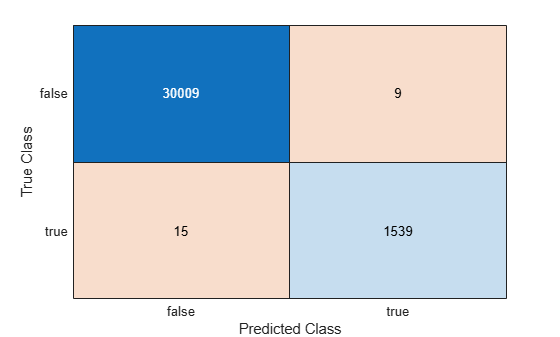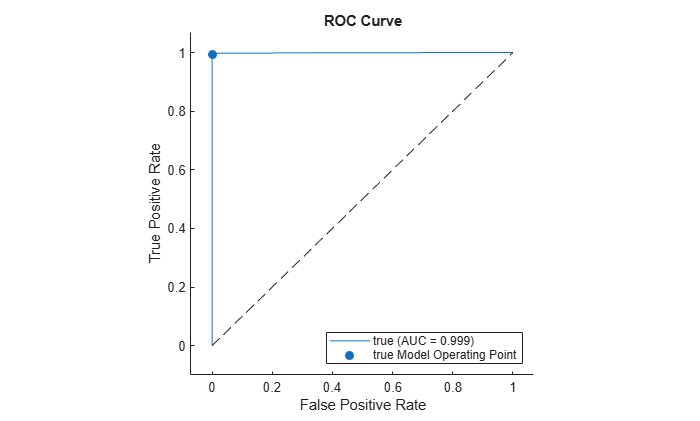# kfoldPredict

Predict labels for observations not used for training

## Syntax

``Label = kfoldPredict(CVMdl)``
``````[Label,Score] = kfoldPredict(CVMdl)``````

## Description

example

````Label = kfoldPredict(CVMdl)` returns cross-validated class labels predicted by the cross-validated, binary, linear classification model `CVMdl`. That is, for every fold, `kfoldPredict` predicts class labels for observations that it holds out when it trains using all other observations.`Label` contains predicted class labels for each regularization strength in the linear classification models that compose `CVMdl`.```

example

``````[Label,Score] = kfoldPredict(CVMdl)``` also returns cross-validated classification scores for both classes. `Score` contains classification scores for each regularization strength in `CVMdl`.```

## Input Arguments

expand all

Cross-validated, binary, linear classification model, specified as a `ClassificationPartitionedLinear` model object. You can create a `ClassificationPartitionedLinear` model using `fitclinear` and specifying any one of the cross-validation, name-value pair arguments, for example, `CrossVal`.

To obtain estimates, kfoldPredict applies the same data used to cross-validate the linear classification model (`X` and `Y`).

## Output Arguments

expand all

Cross-validated, predicted class labels, returned as a categorical or character array, logical or numeric matrix, or cell array of character vectors.

In most cases, `Label` is an n-by-L array of the same data type as the observed class labels (see `Y`) used to create `CVMdl`. (The software treats string arrays as cell arrays of character vectors.) n is the number of observations in the predictor data (see `X`) and L is the number of regularization strengths in `CVMdl.Trained{1}.Lambda`. That is, `Label(i,j)` is the predicted class label for observation `i` using the linear classification model that has regularization strength `CVMdl.Trained{1}.Lambda(j)`.

If `Y` is a character array and L > 1, then `Label` is a cell array of class labels.

Cross-validated classification scores, returned as an n-by-2-by-L numeric array. n is the number of observations in the predictor data that created `CVMdl` (see `X`) and L is the number of regularization strengths in `CVMdl.Trained{1}.Lambda`. `Score(i,k,j)` is the score for classifying observation `i` into class `k` using the linear classification model that has regularization strength `CVMdl.Trained{1}.Lambda(j)`. `CVMdl.ClassNames` stores the order of the classes.

If `CVMdl.Trained{1}.Learner` is `'logistic'`, then classification scores are posterior probabilities.

## Examples

expand all

`load nlpdata`

`X` is a sparse matrix of predictor data, and `Y` is a categorical vector of class labels. There are more than two classes in the data.

The models should identify whether the word counts in a web page are from the Statistics and Machine Learning Toolbox™ documentation. So, identify the labels that correspond to the Statistics and Machine Learning Toolbox™ documentation web pages.

`Ystats = Y == 'stats';`

Cross-validate a binary, linear classification model using the entire data set, which can identify whether the word counts in a documentation web page are from the Statistics and Machine Learning Toolbox™ documentation.

```rng(1); % For reproducibility CVMdl = fitclinear(X,Ystats,'CrossVal','on'); Mdl1 = CVMdl.Trained{1}```
```Mdl1 = ClassificationLinear ResponseName: 'Y' ClassNames: [0 1] ScoreTransform: 'none' Beta: [34023x1 double] Bias: -1.0008 Lambda: 3.5193e-05 Learner: 'svm' Properties, Methods ```

`CVMdl` is a `ClassificationPartitionedLinear` model. By default, the software implements 10-fold cross validation. You can alter the number of folds using the `'KFold'` name-value pair argument.

Predict labels for the observations that `fitclinear` did not use in training the folds.

`label = kfoldPredict(CVMdl);`

Because there is one regularization strength in `Mdl1`, `label` is a column vector of predictions containing as many rows as observations in `X`.

Construct a confusion matrix.

`ConfusionTrain = confusionchart(Ystats,label);`The model misclassifies 15 `'stats'` documentation pages as being outside of the Statistics and Machine Learning Toolbox documentation, and misclassifies nine pages as `'stats'` pages.

Linear classification models return posterior probabilities for logistic regression learners only.

Load the NLP data set and preprocess it as in Predict k-fold Cross-Validation Labels. Transpose the predictor data matrix.

```load nlpdata Ystats = Y == 'stats'; X = X';```

Cross-validate binary, linear classification models using 5-fold cross-validation. Optimize the objective function using SpaRSA. Lower the tolerance on the gradient of the objective function to `1e-8`.

```rng(10); % For reproducibility CVMdl = fitclinear(X,Ystats,'ObservationsIn','columns',... 'KFold',5,'Learner','logistic','Solver','sparsa',... 'Regularization','lasso','GradientTolerance',1e-8);```

Predict the posterior class probabilities for observations not used to train each fold.

```[~,posterior] = kfoldPredict(CVMdl); CVMdl.ClassNames```
```ans = 2x1 logical array 0 1 ```

Because there is one regularization strength in `CVMdl`, `posterior` is a matrix with 2 columns and rows equal to the number of observations. Column i contains posterior probabilities of `Mdl.ClassNames(i)` given a particular observation.

Obtain false and true positive rates, and estimate the AUC. Specify that the second class is the positive class.

```[fpr,tpr,~,auc] = perfcurve(Ystats,posterior(:,2),CVMdl.ClassNames(2)); auc```
```auc = 0.9989 ```

The AUC is `0.9990`, which indicates a model that predicts well.

Plot an ROC curve.

```figure; plot(fpr,tpr) h = gca; h.XLim(1) = -0.1; h.YLim(2) = 1.1; xlabel('False positive rate') ylabel('True positive rate') title('ROC Curve')```The ROC curve indicates that the model classifies almost perfectly.

To determine a good lasso-penalty strength for a linear classification model that uses a logistic regression learner, compare cross-validated AUC values.

Load the NLP data set. Preprocess the data as in Estimate k-fold Cross-Validation Posterior Class Probabilities.

```load nlpdata Ystats = Y == 'stats'; X = X';```

There are 9471 observations in the test sample.

Create a set of 11 logarithmically-spaced regularization strengths from $1{0}^{-6}$ through $1{0}^{-0.5}$.

`Lambda = logspace(-6,-0.5,11);`

Cross-validate a binary, linear classification models that use each of the regularization strengths and 5-fold cross-validation. Optimize the objective function using SpaRSA. Lower the tolerance on the gradient of the objective function to `1e-8`.

```rng(10); % For reproducibility CVMdl = fitclinear(X,Ystats,'ObservationsIn','columns',... 'KFold',5,'Learner','logistic','Solver','sparsa',... 'Regularization','lasso','Lambda',Lambda,'GradientTolerance',1e-8)```
```CVMdl = classreg.learning.partition.ClassificationPartitionedLinear CrossValidatedModel: 'Linear' ResponseName: 'Y' NumObservations: 31572 KFold: 5 Partition: [1×1 cvpartition] ClassNames: [0 1] ScoreTransform: 'none' Properties, Methods ```
`Mdl1 = CVMdl.Trained{1}`
```Mdl1 = ClassificationLinear ResponseName: 'Y' ClassNames: [0 1] ScoreTransform: 'logit' Beta: [34023×11 double] Bias: [-13.2904 -13.2904 -13.2904 -13.2904 -9.9357 -7.0782 -5.4335 -4.5473 -3.4223 -3.1649 -2.9795] Lambda: [1.0000e-06 3.5481e-06 1.2589e-05 4.4668e-05 1.5849e-04 5.6234e-04 0.0020 0.0071 0.0251 0.0891 0.3162] Learner: 'logistic' Properties, Methods ```

`Mdl1` is a `ClassificationLinear` model object. Because `Lambda` is a sequence of regularization strengths, you can think of `Mdl1` as 11 models, one for each regularization strength in `Lambda`.

Predict the cross-validated labels and posterior class probabilities.

```[label,posterior] = kfoldPredict(CVMdl); CVMdl.ClassNames; [n,K,L] = size(posterior)```
```n = 31572 ```
```K = 2 ```
```L = 11 ```
`posterior(3,1,5)`
```ans = 1.0000 ```

`label` is a 31572-by-11 matrix of predicted labels. Each column corresponds to the predicted labels of the model trained using the corresponding regularization strength. `posterior` is a 31572-by-2-by-11 matrix of posterior class probabilities. Columns correspond to classes and pages correspond to regularization strengths. For example, `posterior(3,1,5)` indicates that the posterior probability that the first class (label `0`) is assigned to observation 3 by the model that uses `Lambda(5)` as a regularization strength is 1.0000.

For each model, compute the AUC. Designate the second class as the positive class.

```auc = 1:numel(Lambda); % Preallocation for j = 1:numel(Lambda) [~,~,~,auc(j)] = perfcurve(Ystats,posterior(:,2,j),CVMdl.ClassNames(2)); end```

Higher values of `Lambda` lead to predictor variable sparsity, which is a good quality of a classifier. For each regularization strength, train a linear classification model using the entire data set and the same options as when you trained the model. Determine the number of nonzero coefficients per model.

```Mdl = fitclinear(X,Ystats,'ObservationsIn','columns',... 'Learner','logistic','Solver','sparsa','Regularization','lasso',... 'Lambda',Lambda,'GradientTolerance',1e-8); numNZCoeff = sum(Mdl.Beta~=0);```

In the same figure, plot the test-sample error rates and frequency of nonzero coefficients for each regularization strength. Plot all variables on the log scale.

```figure; [h,hL1,hL2] = plotyy(log10(Lambda),log10(auc),... log10(Lambda),log10(numNZCoeff + 1)); hL1.Marker = 'o'; hL2.Marker = 'o'; ylabel(h(1),'log_{10} AUC') ylabel(h(2),'log_{10} nonzero-coefficient frequency') xlabel('log_{10} Lambda') title('Cross-Validated Statistics') hold off```Choose the index of the regularization strength that balances predictor variable sparsity and high AUC. In this case, a value between $1{0}^{-3}$ to $1{0}^{-1}$ should suffice.

`idxFinal = 9;`

Select the model from `Mdl` with the chosen regularization strength.

`MdlFinal = selectModels(Mdl,idxFinal);`

`MdlFinal` is a `ClassificationLinear` model containing one regularization strength. To estimate labels for new observations, pass `MdlFinal` and the new data to `predict`.